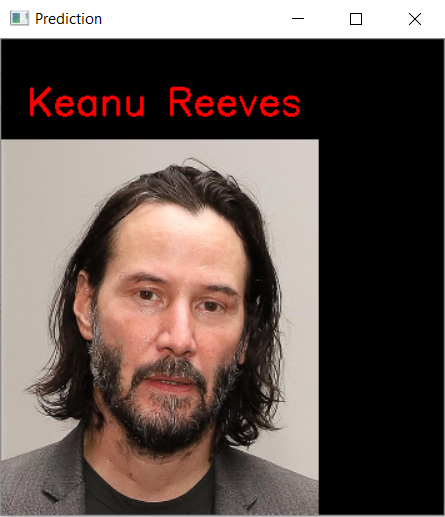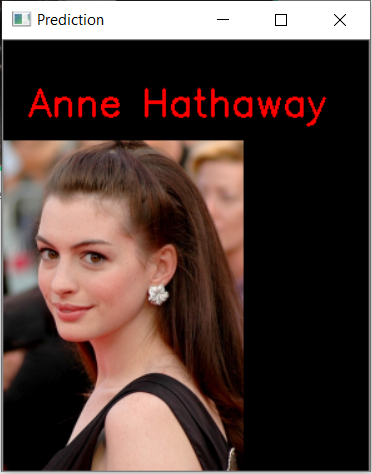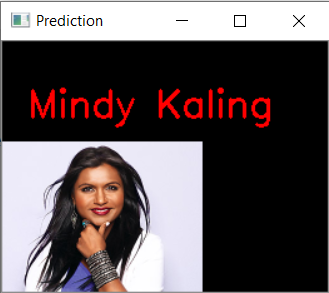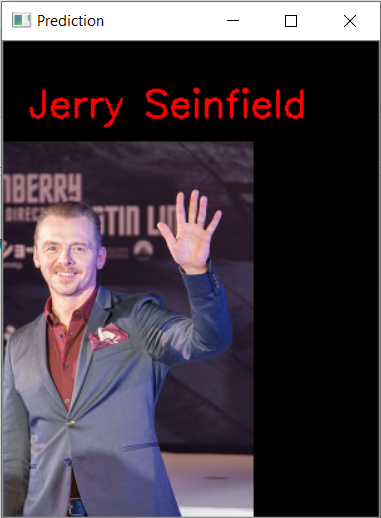# An Introduction to Transfer Learning

Machine Learning and Deep Learning are vast fields with a wide range of applications ranging from image recognition, speech recognition, recommendation and association systems, etc. To build any model from scratch, we require a lot of storage and computing power, which may not always be available to us. Also, we can face situations where we have methods to improve existing models, but the complications of training the models from scratch once again prevent us from doing so. To handle such objectives, we can use the concept of Transfer Learning.

Transfer learning is a machine learning method where a model developed for a task is reused as the starting point for a model on a second task. In this method, pre-trained models are used as the starting point on computer vision and natural language processing tasks instead of developing models from the very beginning. This allows us to handle the challenge of the large amount of computing and storage resources required to develop Deep Learning models. However, it should also be noted that transfer learning only works in deep learning if the model features learned from the first task are general.

In this article, we can see an implementation of transfer learning for face recognition using the MobileNet architecture. The pre-trained weights for MobileNet can be found in Keras and downloaded. There are other Neural Network architectures like VGG16, VGG19, ResNet50, Inception V3, etc, but MobileNet comes with its own set of advantages:

1. It is lightweight and can be used for embedded and mobile applications.
2. There are a reduced number of parameters.
3. It is a low-latency neural network.

MobileNet also comes with a small disadvantage of reduced accuracy due to the lower size, the number of parameters, and preference of higher performance speed.

The dataset being used here is based on the 14 Celebrity dataset taken from Kaggle, one of the most popular websites for working with Machine Learning and Deep Learning problems and getting datasets. In addition to the data already present, I have added more images from my end from Google Images, to bring a total of 283 training images and 70 test images spread across 14 classes.

To implement MobileNet, we first download the weights of the pre-trained MobileNet architecture from Keras. In transfer learning, we freeze the previously trained layers and add new layers to the existing model to develop a newer and improved model tailored to our requirements.

`from keras.applications import MobileNet# MobileNet was designed to work on 224 x 224 pixel input images sizesimg_rows, img_cols = 224, 224# Re-loads the MobileNet model without the top or FC layersMobileNet = MobileNet(weights = 'imagenet',                  include_top = False,                  input_shape = (img_rows, img_cols, 3)) # Layers are set to trainable as True by defaultfor layer in MobileNet.layers:    layer.trainable = False    # Printing layers for (i,layer) in enumerate(MobileNet.layers):    print(str(i) + " "+ layer.__class__.__name__, layer.trainable)`

To add new layers, we create a function that we can call later under the name `get_head` . Here, we retrieve the layers of the MobileNet model and add new layers (One Pooling Layer and 4 Dense Layers. The final Dense layer is for prediction).

`def get_head(bottom_model, num_classes):    """creates the top or head of the model that will be     placed ontop of the bottom layers"""top_model = bottom_model.output    top_model = GlobalAveragePooling2D()(top_model)    top_model = Dense(1024,activation='relu')(top_model)    top_model = Dense(512,activation='relu')(top_model)    top_model = Dense(512,activation='relu')(top_model)    top_model = Dense(num_classes,activation='softmax')(top_model)    return top_model`

Next, we import the required modules for adding layers and call the function to add the new layers. Currently, we are using

`from keras.models import Sequentialfrom keras.layers import Dense, Dropout, Activation, Flatten, GlobalAveragePooling2Dfrom keras.layers import Conv2D, MaxPooling2D, ZeroPadding2Dfrom keras.layers.normalization import BatchNormalizationfrom keras.models import Model# Number of new classes for training the model = 14num_classes = 14FC_Head = get_head(MobileNet, num_classes)model = Model(inputs = MobileNet.input, outputs = FC_Head)print(model.summary())`

Image processing applications in Machine Learning and Deep Learning require a vast amount of training and testing data. If we are not able to collect enough data, we can use the ImageDataGenerator offered by Keras to expand our current image dataset. The ImageDataGenerator is typically used to perform operations like rotation, horizontal flips, vertical flips et. on images to bring more variation into the training and testing data. This helps in building a good model.

`from keras.preprocessing.image import ImageDataGeneratortrain_data_dir = 'D://Online Courses//MLOps//Notes from MLOps Drive//Transfer Learning//dataset//train//'validation_data_dir = 'D://Online Courses//MLOps//Notes from MLOps Drive//Transfer Learning//dataset//test//'# Let's use some data augmentaiton train_datagen = ImageDataGenerator(      rescale=1./255,      rotation_range=45,      width_shift_range=0.3,      height_shift_range=0.3,      horizontal_flip=True,      fill_mode='nearest') validation_datagen = ImageDataGenerator(rescale=1./255) # set our batch size (typically on most mid tier systems we'll use 16-32)batch_size = 32 train_generator = train_datagen.flow_from_directory(        train_data_dir,        target_size=(img_rows, img_cols),        batch_size=batch_size,        class_mode='categorical') validation_generator = validation_datagen.flow_from_directory(        validation_data_dir,        target_size=(img_rows, img_cols),        batch_size=batch_size,        class_mode='categorical')`

Now, we have the training and testing data ready for use. We can proceed with the model training. We can use the concept of checkpoints, Early Stops, and callbacks to make our training process more efficient. The trained model is stored in the file `face_recognition.h5` . To test our model, we can import the trained weights from this file.

`from keras.optimizers import RMSpropfrom keras.callbacks import ModelCheckpoint, EarlyStoppingcheckpoint = ModelCheckpoint("face_recognition.h5",                             monitor="val_loss",                             mode="min",                             save_best_only = True,                             verbose=1)earlystop = EarlyStopping(monitor = 'val_loss',                           min_delta = 0,                           patience = 3,                          verbose = 1,                          restore_best_weights = True)# we put our call backs into a callback listcallbacks = [earlystop, checkpoint]# We use a very small learning rate model.compile(loss = 'categorical_crossentropy',              optimizer = RMSprop(lr = 0.001),              metrics = ['accuracy'])# Enter the number of training and validation samples herenb_train_samples = 2100nb_validation_samples = 140# We only train 5 EPOCHS epochs = 5batch_size = 16history = model.fit_generator(    train_generator,    steps_per_epoch = nb_train_samples // batch_size,    epochs = epochs,    callbacks = callbacks,    validation_data = validation_generator,    validation_steps = nb_validation_samples // batch_size)`

Now, we test our model with any given image. I have taken one image from the testing data. In the code, the final output is the image being tested for prediction, with the predicted class displayed on the image window as well. For displaying the image, we use the cv2 module in python.

`import osimport cv2import numpy as npfrom os import listdirfrom os.path import isfile, joinfrom keras.models import load_modelclassifier = load_model('face_recognition.h5')faces_dict = {"": "Anne Hathaway",               "": "Arnold Schwarzenegger",              "": "Ben Afflek",              "": "Dwayne Johnson",              "": "Elton John",              "": "Jerry Seinfield",              "": "Kate Beckinsale",              "": "Keanu Reeves",              "": "Lauren Cohan",              "": "Madonna",              "": "Mindy Kanling",              "": "Simon Pegg",              "": "Sofia Vergara",              "": "Will Smith"}faces_dict_n = {"n0": "Anne Hathaway",                 "n1": "Arnold Schwarzenegger",                "n2": "Ben Afflek",                "n3": "Dwayne Johnson",                "n4": "Elton John",                "n5": "Jerry Seinfield",                "n6": "Kate Beckinsale",                "n7": "Keanu Reeves",                "n8": "Lauren Cohan",                "n9": "Madonna",                "n10": "Mindy Kanling",                "n11": "Simon Pegg",                "n12": "Sofia Vergara",                "n13": "Will Smith"               }def draw_test(name, pred, im):    face_img = faces_dict[str(pred)]    BLACK = [0,0,0]    expanded_image = cv2.copyMakeBorder(im, 80, 0, 0, 100 ,cv2.BORDER_CONSTANT,value=BLACK)    cv2.putText(expanded_image, face_img, (20, 60) , cv2.FONT_HERSHEY_SIMPLEX,1, (0,0,255), 2)    cv2.imshow(name, expanded_image)input_im = cv2.imread('D://Online Courses//MLOps//Notes from MLOps Drive//Transfer Learning//dataset//testimg_1.jpg')input_original = input_im.copy()input_original = cv2.resize(input_original, None, fx=0.5, fy=0.5, interpolation = cv2.INTER_LINEAR)    input_im = cv2.resize(input_im, (224, 224), interpolation = cv2.INTER_LINEAR)input_im = input_im / 255.input_im = input_im.reshape(1,224,224,3)     # Get Predictionresult = np.argmax(classifier.predict(input_im, 1, verbose = 0), axis=1)# Show image with predicted classimport cv2cap = cv2.VideoCapture(0)draw_test("Prediction", result, input_original) cv2.waitKey(0)cv2.destroyAllWindows()`

OutputsFigure 1: Actual Image — Keanu Reeves; Prediction — Keanu ReevesFigure 2: Actual Image — Anne Hathaway; Prediction — Anne HathawayFigure 3: Actual Image — Mindy Kaling; Prediction — Mindy KalingFigure 4: Actual Image — Lauren Cohan; Prediction — Anna HathawayFigure 5: Actual Image — Simon Pegg; Prediction — Jerry Seinfield

From the results above, we can see that the model doesn't always give the correct answer. Such errors are often due to training dataset size and variation and the hyperparameters used. By varying different hyperparameters like `batch size`, and increasing the volume and variation of training data, we can build better models that give greater accuracy.

References

1. https://machinelearningmastery.com/transfer-learning-for-deep-learning/
2. Research Paper where the MobileNet architecture was published — A. G. Howard, M. Zhu, B. Chen, D. Kalenichenko, W. Wang, T. Weyand, M. Andreetto, and H. Adam. Mobilenets: Efficient convolutional neural networks for mobile vision applications. arXiv:1704.04861, 2017.